Predicted Values of the Mean

### Predicted Values

A predicted value, or fitted value, of the mean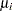corresponding to the vector of covariates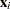is given by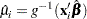whereis the link function, regardless of whethercorresponds to an observation or not. That is, the response variable can be missing and the predicted value is still computed for valid. In the case wheredoes not correspond to a valid observation,is not checked for estimability. You should check the estimability ofin this case in order to ensure the uniqueness of the predicted value of the mean. If there is an offset, it is included in the predicted value computation.

### Confidence Intervals on Predicted Values

Approximate confidence intervals for predicted values of the mean can be computed as follows. The variance of the linear predictor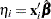is estimated by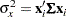where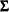is the estimated covariance of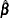. The robust estimate of the covariance is used forin the case of models fit with GEEs.

Approximate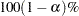confidence intervals are computed as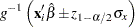where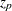is the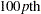percentile of the standard normal distribution andis the link function. If either endpoint in the argument is outside the valid range of arguments for the inverse link function, the corresponding confidence interval endpoint is set to missing.